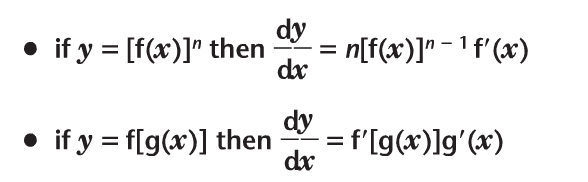# Very simple exponential function quesiton

phospho
The derivative of e^(2x):

let y = e^(2x), let u = 2x, so y = e^u

chain rule: du/dx * dy/du = 2*e^u = 2e^u = 2e^(2x)

this is the solution copied from my book, my question is why do they let u = 2x? is e^u the same as e^x? If so then wouldn't all derivatives of the exponential functions be in the form of f`(x)e^(f(x))??

Yes, the derivative of e^(f(x)) is f'(x) e^(f(x)), regardless of what f(x) is. Does that answer your question?

phospho
Yes, the derivative of e^(f(x)) is f'(x) e^(f(x)), regardless of what f(x) is. Does that answer your question?
Why in the solutions do they let u = 2x? Is differentiating e^u the same as differentiating e^x?

Staff Emeritus
Homework Helper
You have to keep in mind which variable you are differentiating with respect to.
\begin{align*}
\frac{d}{du} e^u &= e^u \\
\frac{d}{dx} e^u &= e^u\frac{du}{dx}
\end{align*}

Homework Helper
Dearly Missed
Why in the solutions do they let u = 2x? Is differentiating e^u the same as differentiating e^x?

They are just using (d/dx) e^f(x) = f'(x) e^f(x) [an equation which YOU wrote!], with f(x) = 2x.

RGV

phospho
You have to keep in mind which variable you are differentiating with respect to.
\begin{align*}
\frac{d}{du} e^u &= e^u \\
\frac{d}{dx} e^u &= e^u\frac{du}{dx}
\end{align*}

thanks for all the replies, how did you figure out the second line of your reply? (differentiating e^u with respect to x) as I don't think I've seen that before (I've only just started calc).

Staff Emeritus
Homework Helper
It's just the chain rule.

phospho
It's just the chain rule.

My book says this about the chain rule:How does what you said about differentiating e^u with respect to x apply to what my book said? Could you explain further as I'm confused.

1MileCrash
Because u is a function of x.

So e^u's derivative, by the chain rule, is u'e^u.

Staff Emeritus
Homework Helper
Can you explain what's confusing you? I mean, we can keep telling you "It's the chain rule," but it's hard to see what's troubling you.

phospho
Can you explain what's confusing you? I mean, we can keep telling you "It's the chain rule," but it's hard to see what's troubling you.

I just don't really understand the chain rule.

Perhaps it would help to write u as u(x). Then can you see that the chain rule gives:
$$\frac{d}{dx}e^{u(x)} =e^{u(x)}\frac{du}{dx}$$

If u is not a function of x, then:
$$\frac{d}{dx}e^{u} =0$$

Does this help?

1MileCrash
I just don't really understand the chain rule.

In my opinion, written out, it looks much more complicated than it is.

Take the derivative of the outer function, still evaluated for the inner function, then multiply the whole thing by the derivative of the inner function.

One thing that is cool about the derivative rules is that they always work. For example, take a function that you know the derivative of, like 2x. You can apply the chain rule, product rule, etc. to that and you will get the correct result.

2(x).

Derivative of the outer function, evaluated for x, multiplied by the derivative of x, is just 2(1), or 2.

phospho
Perhaps it would help to write u as u(x). Then can you see that the chain rule gives:
$$\frac{d}{dx}e^{u(x)} =e^{u(x)}\frac{du}{dx}$$

If u is not a function of x, then:
$$\frac{d}{dx}e^{u} =0$$

Does this help?

Not really, I don't understand if u is not a function of x then that makes the derivative = 0,

In my opinion, written out, it looks much more complicated than it is.

Take the derivative of the outer function, still evaluated for the inner function, then multiply the whole thing by the derivative of the inner function.

One thing that is cool about the derivative rules is that they always work. For example, take a function that you know the derivative of, like 2x. You can apply the chain rule, product rule, etc. to that and you will get the correct result.

2(x).

Derivative of the outer function, evaluated for x, multiplied by the derivative of x, is just 2(1), or 2.

Yes, but I don't really see how this applies when differentiating with respect to another variable as above

thank you for everyone who has bared with me, I'll probably sleep as I just don't understand it at all.

1MileCrash
Yes, but I don't really see how this applies when differentiating with respect to another variable as above

thank you for everyone who has bared with me, I'll probably sleep as I just don't understand it at all.

Put it this way:

If I say u = 2x, then I ask you to find the derivatives of e^(2x) and e^(u), you had better get the same answer.

If you apply the chain rule to e^(2x) and not to e^(u) just because u is a "single letter," you won't get the same result.

Not really, I don't understand if u is not a function of x then that makes the derivative = 0,

What's the derivative of e^(6)?

Last edited: